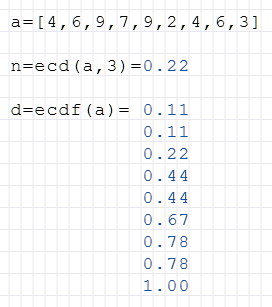# Ecd Function

Calculation of the empirical distribution of a set

## Description

The function $$Ecd$$ (Empirical Cumulative Distribution) calculates the empirical distribution of a set. The Empirical Cumulative Distribution is the empirical distribution function that gives each number in the list the proportion of sample values, which are smaller or equal, assigns.

The result is the value at the specified index.

#### Syntax

Ecd(list) = list

Ecd(list, index) = value

#### Example